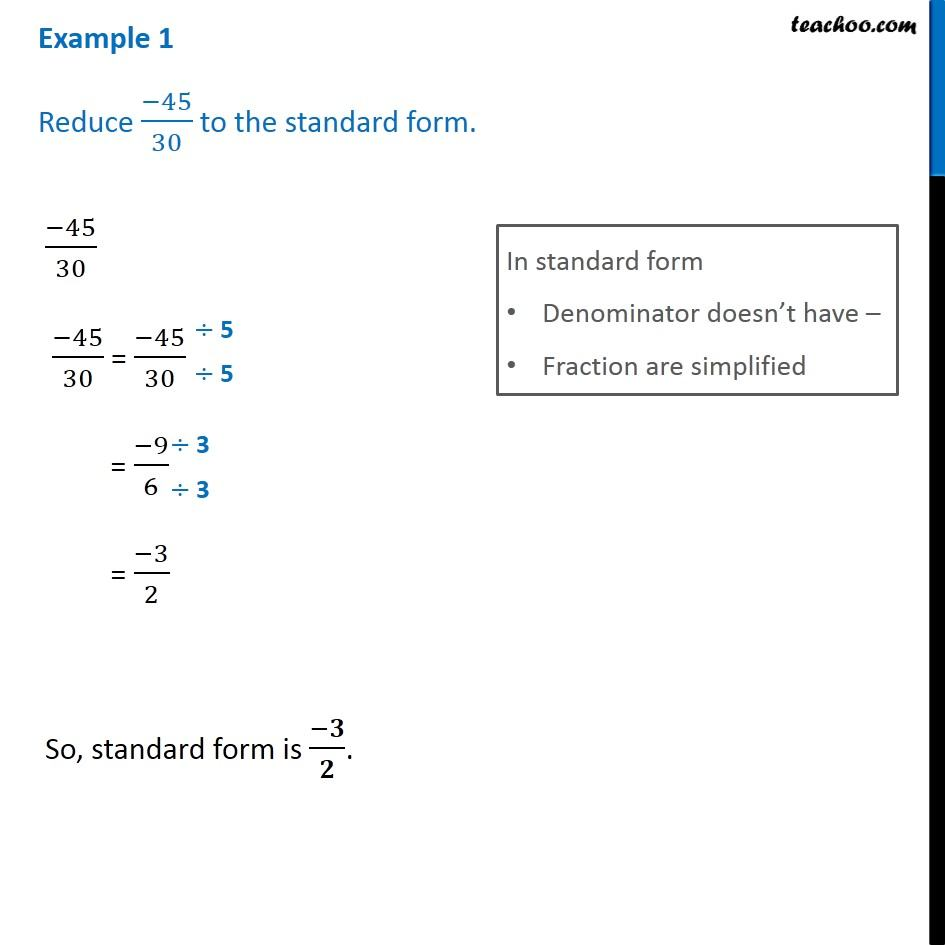Examples

Chapter 9 Class 7 Rational Numbers
Serial order wise### Transcript

Example 1 Reduce (−45)/30 to the standard form. (−45)/30 (−45)/30 = (−45)/30 In standard form Denominator doesn’t have – Fraction are simplified = (−9)/6 = (−3)/2 So, standard form is (−𝟑)/𝟐.